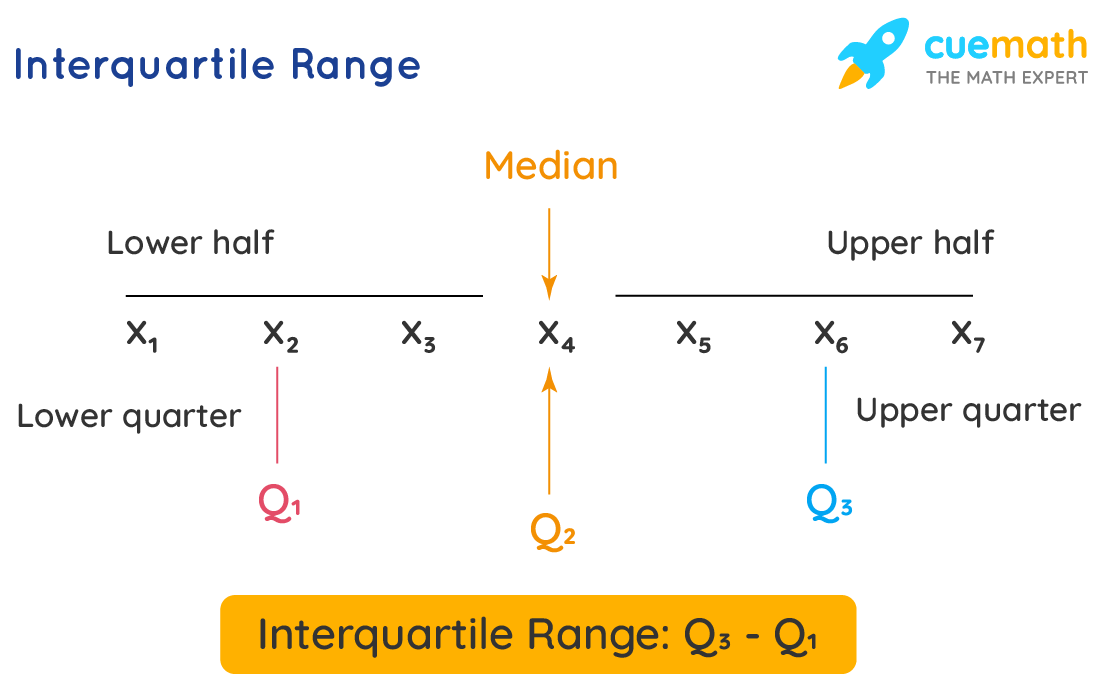# Interquartile Range

The smallest of all the measures of dispersion in statistics is called the Interquartile Range. Interquartile Range is the difference between the two extreme observations or terms of the distribution.

In Interquartile Range quartiles are the partitional values that divide the whole number series into 4 equal parts. These quartiles are represented by Q1( the lower quartile), Q2 (the median), and Q3 (the upper quartile).

Therefore, the difference between the upper and lower quartile is known as the interquartile range.

## What is Interquartile Range?

The Interquartile Range can be given as:

Interquartile range = Upper Quartile – Lower Quartile = Q­3 – Q­1where,

Q1 is the first quartile and Q3 is the third quartile of the series

Q2 is the second quartile or median of the series.

Half of the interquartile range formula is given by:

Semi Interquartile Range = (Q3– Q1) / 2

Few steps to calculate the interquartile range:

• Arrange the number series into increasing or decreasing order.
• If the number series is odd, then the center value is median otherwise for even number series the median is the mean value for two center values.
• The median of the given number series equally cuts the given values into two equal parts, we refer to this as Q2.
• Qcan be calculated using the formula:

Q1 = $$\dfrac{(n+1)}{4}^{\text{th}}$$ term

• Q3 can be calculated using the formula:

Q3 = $$\dfrac{3(n+1)}{4}^{\text{th}}$$ term

where,

n = Number of terms

• Finally, we have to subtract the median values of Q1 and Q3. The resulting value is the interquartile range of series.

## Solved Examples Using Interquartile Range

### Determine the interquartile range value for the first ten odd numbers.

Solution:

To find: The interquartile range value for the first ten odd numbers

Given:

The first ten odd numbers:1, 3, 5, 7, 9, 11, 13, 15, 17, 18

Number of values = 10

8 is an even number. Therefore, the median is the mean of 5th and 6th terms.

That is Q2 = (9+11)/2.

Q2 = 10.

Now

Q1 part is {1, 3, 5, 7, 9}

Here the number of values = 5

5 is an odd number. Therefore, the center value Q1 is  5

Q3 part : {11, 13, 15, 17, 19}

Here the number of values = 5

5 is an odd number. Therefore, the center value Q3 is 15

Using Interquartile Range,

Interquartile Range = Upper Quartile – Lower Quartile = Q­3 – Q­1

Q3 - Q1 is 15 – 5 = 10

Answer: 15 is the interquartile range for the given set of first 10 odd numbers.

### {4, 17, 7, 14, 18, 12, 3, 16, 10, 4, 4, 11}

Solution:

To find: Interquartile range

Given:

Number of terms = 12

Set = {4, 17, 7, 14, 18, 12, 3, 16, 10, 4, 4, 11}

Ordered set = {3, 4, 4, 4, 7, 10, 11, 12, 14, 16, 17, 18}

Dividing the set into quartiles, each quarter will have 3 terms as: {3, 4, 4}, {4, 7, 10}, {11, 12, 14}, {16, 17, 18}

First Quartile,

Q1 = (4 + 4)/ 2 = 4

Third Quartile,

Q3 = (14 + 16)/2 = 15

Using Interquartile Range Formula,

Interquartile Range = Upper Quartile – Lower Quartile = Q­3 – Q­1

= 15 - 4

= 11

Answer: Interquartile range of the given set = 11